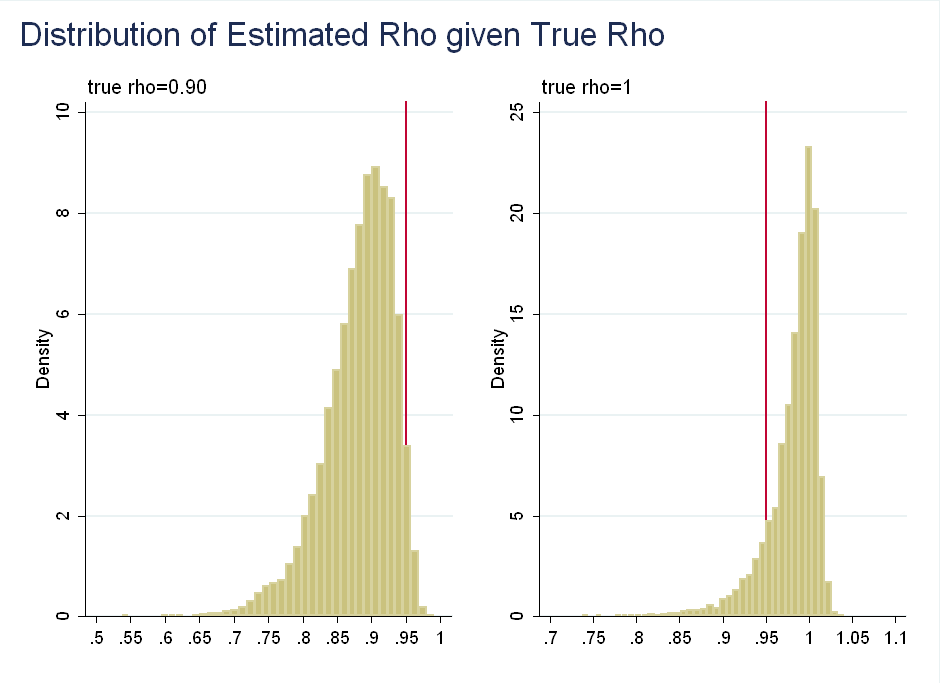Sims and Uhlig argue: although classical (frequentist) $p$-values are asymptotically equivalent to Bayesian posteriors, they should not be interpreted as probabilities. This is because the equivalence breaks down in non-stationary models.
The paper uses small sample sizes, with $T=100$ - This post examines how the results change with $T=10,000$, when the asymptotic behavior kicks in.

# The Setup

Consider a simpler AR(1) model: \begin{equation} y_t=\rho y_{t-1} + \epsilon_t \end{equation} To simplify things, suppose $\epsilon_t \sim N(0,1)$. Classical inference suggests that for $% $, the estimator is asymptotically normal and converges at rate $\sqrt{T}$: \begin{equation} \sqrt{T}(\hat{\rho}-\rho) \rightarrow^{L} N(0,(1-\rho^2)) \end{equation} For $\rho=1$, however, we get a totally different distribution, which converges at rate $T$, instead of rate $\sqrt{T}$: \begin{equation} T(\hat{\rho}-\rho)=T(\hat{\rho}-1)= \rightarrow^{L} \frac{(1/2)([W(1)]^2-1)}{\int_0^1 [W(r)]^2 dr} \end{equation} where $W(1)$ is a Brownian motion. Although it looks complicated, it is easier to visualize when you see $W(1)^2$ is actually a $\chi^2(1)$ variable. This is left skewed, as the probability that a $\chi^2(1)$ is less than one is 0.68 and large realizations of $[W(1)]^2$ in the numerator get down-weighted by a large denominator (it is the same Brownian motion in the numerator and denominator). In the paper, the authors choose 31 values of $\rho$ between 0.8 to 1.1 in increments of 0.01. For each $\rho$ they simulate 10,000 samples of the AR(1) model described above with $T=100$. Finally, they run an OLS regression of $y_t$ on $y_{t-1}$ to get the distributions for $\hat{\rho}$ (the OLS estimator of $\rho$). Below I show the distribution of $\hat{\rho}$ for selected values of $\rho$:Another way to think about the data is to look at the distribution of $\rho$ given observed values of $\hat{\rho}$. This is symmetric about 0.95:Their problem with using $p$-values as probabilities is that if we observe $\hat{\rho}=0.95$, we can reject the null of $\rho=0.9$, but we fail to reject the null of $\rho=1$ (think about the area in the tails after normalizing the distribution to integrate to 1), even though $\rho$ given $\hat{\rho}$ is roughly symmetric about 0.95:The problem is distortion by irrelevant information: Values of $\hat{\rho}$ much below 0.95 are more likely given $\rho=1$ than are values of $\hat{\rho}$ much above 0.95 given $\rho=0.9$. This is irrelevant as we have already observed $\hat{\rho}=0.95$, so we know it is not far above or below.

The prior required to generate these results (i.e. the prior that would let us interpret $p$-values as posterior probabilities) is sample dependent. Usually, classical inference is asymptotically equivalent to Bayesian inference using a flat prior, but it is not the case here. The authors show that classical analysis is implicitly putting progressively more weight on values of $\rho$ above one as $\hat{\rho}$ gets closer to 1.

# Testing with Larger Samples

At first, I found the results counter-intuitive. The first figure above shows that the skewness arrives gradually in finite samples. This is strange, because the asymptotic properties of $\hat{\rho}$ are only non-normal for $\rho=1$. I figured this was the result of using small samples of $T=100$. Under a flat prior, the distribution of $\rho$ given the data and $\epsilon_t$ having variance of 1 is: \begin{equation} \rho \sim N(\hat{\rho}, (\sum\limits_{t=1}^T y_{t-1})^{-1}) \end{equation} This motivates my intuition for why the skewness arrives slowly: even for small samples, as $\rho$ gets close to 1, $\sum\limits_{t=1}^T y_{t-1}$ can be very large.

I repeat their analysis, except instead of $T=100$, I use $T=10,000$. As you can see, the asymptotic behavior kicks in and the skewness arrives only at $\rho=1$:I also found that for $T=10,000$ the distribution of $\rho$ conditional on $\hat{\rho}$, does not spread out more for smaller values of $\hat{\rho}$, that is a small sample result.

# Conclusion

The point of this paper is to show that the classical way of dealing with unit roots implicitly makes undesirable assumptions - you need a sample-dependent prior which puts more weight on high values of $\rho$. To a degree, the authors’ results are driven by the short length of the simulated series. The example where you reject $\rho=0.9$ but fail to reject $\rho=1$ wouldn’t happen in large samples, as the asymptotic kick in and faster rate of convergence for $\rho=1$ gives the distribution less spread.

For now, however, the authors’ criticism is still valid. With quarterly data from 1950-Present you get about 260 observations. Macroeconomics will have to survive until year 4,450 for there to be 10,000 observations, and that’s a long way off.